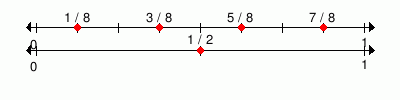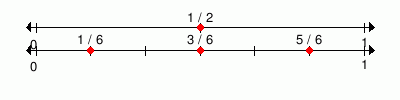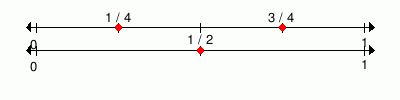MathScore EduFighter is one of the best math games on the Internet today. You can start playing for free!

## Fraction Equivalence - Sample Math Practice Problems

The math problems below can be generated by MathScore.com, a math practice program for schools and individual families. References to complexity and mode refer to the overall difficulty of the problems as they appear in the main program. In the main program, all problems are automatically graded and the difficulty adapts dynamically based on performance. Answers to these sample questions appear at the bottom of the page. This page does not grade your responses.

See some of our other supported math practice problems.

### Complexity=1, Mode=whole

Fill in the blank.

1.
 1 1 1

 31 =

2.
 1 1 1 1 1 1 1 1

 81 =

### Complexity=2, Mode=whole

Fill in the blank.

1.
 41 =

2.
 3 = 3

### Complexity=1, Mode=frac

Fill in the blank.

1.
1
 1/2
 1/8 1/8 1/8 1/8
 12 = 8

2.48 = 1

### Complexity=2, Mode=frac

Fill in the blank.

1.
 12 = 3

2.
 24 = 1

### Complexity=2

Fill in the blank.

1.
 3 = 1

2.
 66 =

### Complexity=1, Mode=whole

Fill in the blank.

1
 1 1 1

 31 =

2
 1 1 1 1 1 1 1 1

 81 =

### Complexity=2, Mode=whole

Fill in the blank.

1
 41 =

Solution
Any number over 1 is equal to itsef.
2
 3 = 3

Solution
Any number over 1 is equal to itsef.

### Complexity=1, Mode=frac

Fill in the blank.

1
1
 1/2
 1/8 1/8 1/8 1/8
 12 = 8

248 = 1

### Complexity=2, Mode=frac

Fill in the blank.

1
 12 = 3

Solution2
 24 = 1

Solution### Complexity=2

Fill in the blank.

1
 3 = 1

Solution
Any number over itself is equal to 1.